# Unimodal distribution¶

The following example demonstrates the statistical learning based determination of the nuclear shielding tensor parameters from a one-dimensional cross-section of a spinning sideband correlation spectrum. In this example, we use a synthetic sideband amplitude spectrum from a unimodal tensor distribution.

## Before getting started¶

Import all relevant packages.

import csdmpy as cp
import matplotlib.pyplot as plt
import numpy as np
from pylab import rcParams

from mrinversion.kernel.nmr import ShieldingPALineshape
from mrinversion.linear_model import SmoothLasso
from mrinversion.linear_model import SmoothLassoCV
from mrinversion.linear_model import TSVDCompression
from mrinversion.utils import get_polar_grids

# Setup for the matplotlib figures
rcParams["figure.figsize"] = 4.5, 3.5
rcParams["font.size"] = 9

# function for 2D x-y plot.
def plot2D(ax, csdm_object, title=""):
# convert the dimension coordinates of the csdm_object from Hz to pmm.
csdm_object.dimensions.to("ppm", "nmr_frequency_ratio")
csdm_object.dimensions.to("ppm", "nmr_frequency_ratio")

levels = (np.arange(9) + 1) / 10
ax.contourf(csdm_object, cmap="gist_ncar", levels=levels)
ax.grid(None)
ax.set_title(title)
get_polar_grids(ax)
ax.set_aspect("equal")


## Dataset setup¶

### Import the dataset¶

Load the dataset. Here, we import the dataset as a CSDM data-object.

# the 1D spinning sideband cross-section data in csdm format
filename = "https://osu.box.com/shared/static/kehokr5op0amkfp5auyd498nblcdr1xy.csdf"

# convert the data dimension from Hz to ppm.
data_object.dimensions.to("ppm", "nmr_frequency_ratio")


The variable data_object holds the 1D dataset. For comparison, let’s also import the true tensor parameter distribution from which the synthetic 1D pure anisotropic spinning sideband cross-section amplitudes is simulated.

datafile = "https://osu.box.com/shared/static/s5wpm26w4cv3w64qjhouqu458ch4z0nd.csdf"


The plot of the 1D sideband cross-section along with the 2D true tensor parameter distribution of the synthetic dataset is shown below.

# the plot of the 1D MAF cross-section dataset.
_, ax = plt.subplots(1, 2, figsize=(9, 3.5), subplot_kw={"projection": "csdm"})
ax.plot(data_object)
ax.invert_xaxis()

# the plot of the true tensor distribution.
plot2D(ax, true_data_object, title="True distribution")
plt.tight_layout()
plt.show()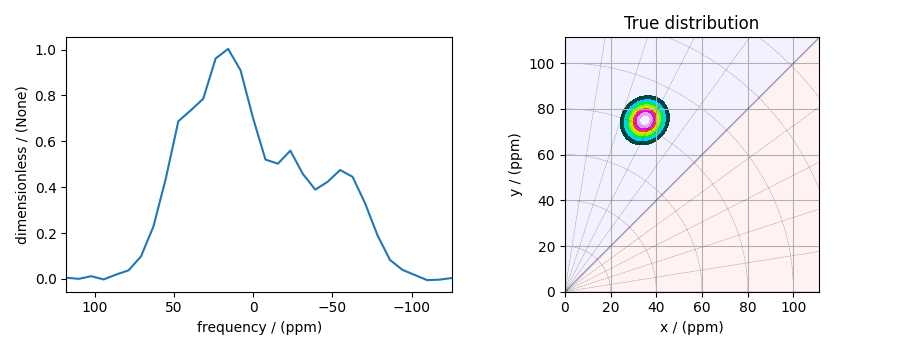## Linear Inversion setup¶

### Dimension setup¶

Anisotropic-dimension: The dimension of the dataset that holds the pure anisotropic spinning sidebands.

anisotropic_dimension = data_object.dimensions


x-y dimensions: The two inverse dimensions corresponding to the x and y-axis of the x-y grid.

inverse_dimension = [
cp.LinearDimension(count=25, increment="370 Hz", label="x"),  # the x-dimension.
cp.LinearDimension(count=25, increment="370 Hz", label="y"),  # the y-dimension.
]


### Generating the kernel¶

For sideband datasets, the line-shape kernel corresponds to the pure anisotropic nuclear shielding spinning sideband spectra. Use the ShieldingPALineshape class to generate the sideband kernel.

lineshape = ShieldingPALineshape(
anisotropic_dimension=anisotropic_dimension,
inverse_dimension=inverse_dimension,
channel="29Si",
magnetic_flux_density="9.4 T",
rotor_angle="54.735 deg",
rotor_frequency="625 Hz",
number_of_sidebands=32,
)


Here, lineshape is an instance of the ShieldingPALineshape class. The required arguments of this class are the anisotropic_dimension, inverse_dimension, and channel. We have already defined the first two arguments in the previous sub-section. The value of the channel argument is the observed nucleus. In this example, this value is ‘29Si’. The remaining arguments, such as the magnetic_flux_density, rotor_angle, and rotor_frequency, are set to match the conditions under which the spectrum was acquired. Note, the rotor frequency is the effective anisotropic modulation frequency, which may be less than the physical rotor frequency. The number of sidebands is usually the number of points along the sideband dimension.

Once the ShieldingPALineshape instance is created, use the kernel() method of the instance to generate the sideband kernel.

K = lineshape.kernel(supersampling=1)


### Data Compression¶

Data compression is optional but recommended. It may reduce the size of the inverse problem and, thus, further computation time.

new_system = TSVDCompression(K, data_object)
compressed_K = new_system.compressed_K
compressed_s = new_system.compressed_s

print(f"truncation_index = {new_system.truncation_index}")


Out:

compression factor = 1.032258064516129
truncation_index = 31


## Solving the inverse problem¶

### Smooth-LASSO problem¶

Solve the smooth-lasso problem. You may choose to skip this step and proceed to the statistical learning method. Usually, the statistical learning method is a time-consuming process that solves the smooth-lasso problem over a range of predefined hyperparameters. If you are unsure what range of hyperparameters to use, you can use this step for a quick look into the possible solution by giving a guess value for the $$\alpha$$ and $$\lambda$$ hyperparameters, and then decide on the hyperparameters range accordingly.

# guess alpha and lambda values.
s_lasso = SmoothLasso(alpha=5e-5, lambda1=5e-6, inverse_dimension=inverse_dimension)
s_lasso.fit(K=compressed_K, s=compressed_s)
f_sol = s_lasso.f


Here, f_sol is the solution corresponding to hyperparameters $$\alpha=5\times10^{-5}$$ and $$\lambda=5\times 10^{-6}$$. The plot of this solution is

_, ax = plt.subplots(1, 2, figsize=(9, 3.5), subplot_kw={"projection": "csdm"})

# the plot of the guess tensor distribution solution.
plot2D(ax, f_sol / f_sol.max(), title="Guess distribution")

# the plot of the true tensor distribution.
plot2D(ax, true_data_object, title="True distribution")
plt.tight_layout()
plt.show()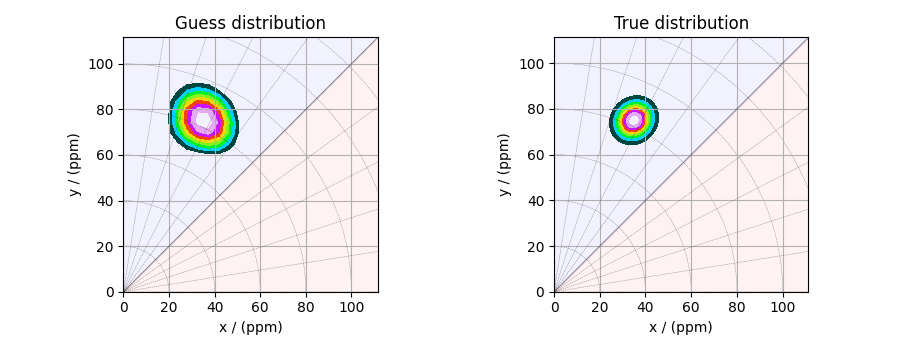### Predicted spectrum¶

You may also evaluate the predicted spectrum from the above solution following

residuals = s_lasso.residuals(K, data_object)
predicted_spectrum = data_object - residuals

plt.figure(figsize=(4, 3))
plt.gca(projection="csdm")
plt.plot(data_object, color="black", label="spectrum")  # the original spectrum
plt.plot(predicted_spectrum, color="red", label="prediction")  # the predicted spectrum
plt.gca().invert_xaxis()
plt.legend()
plt.tight_layout()
plt.show()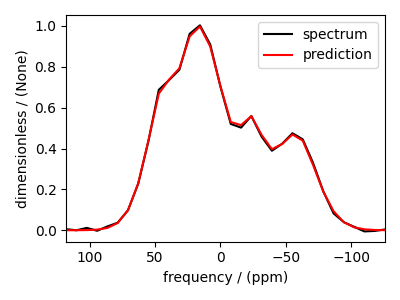As you can see from the predicted spectrum, our guess isn’t far from the optimum hyperparameters. Let’s create a search grid about the guess hyperparameters and run a cross-validation method for selection.

## Statistical learning of the tensors¶

### Smooth LASSO cross-validation¶

Create a guess range of values for the $$\alpha$$ and $$\lambda$$ hyperparameters. The following code generates a range of $$\lambda$$ and $$\alpha$$ values that are uniformly sampled on the log scale.

lambdas = 10 ** (-5 - 1 * (np.arange(6) / 5))
alphas = 10 ** (-4 - 2 * (np.arange(6) / 5))

# set up cross validation smooth lasso method
s_lasso_cv = SmoothLassoCV(
alphas=alphas,
lambdas=lambdas,
inverse_dimension=inverse_dimension,
sigma=0.005,
folds=10,
)
# run the fit using the compressed kernel and compressed signal.
s_lasso_cv.fit(compressed_K, compressed_s)


### The optimum hyper-parameters¶

Use the hyperparameters attribute of the instance for the optimum hyper-parameters, $$\alpha$$ and $$\lambda$$, determined from the cross-validation.

print(s_lasso_cv.hyperparameters)


Out:

{'alpha': 2.5118864315095823e-06, 'lambda': 1.584893192461114e-06}


### The cross-validation surface¶

Optionally, you may want to visualize the cross-validation error curve/surface. Use the cross_validation_curve attribute of the instance, as follows. The cross-validation metric is the mean square error (MSE).

cv_curve = s_lasso_cv.cross_validation_curve

# plot of the cross-validation curve
plt.figure(figsize=(5, 3.5))
ax = plt.subplot(projection="csdm")
ax.contour(np.log10(s_lasso_cv.cross_validation_curve), levels=25)
ax.scatter(
-np.log10(s_lasso_cv.hyperparameters["alpha"]),
-np.log10(s_lasso_cv.hyperparameters["lambda"]),
marker="x",
color="k",
)
plt.show()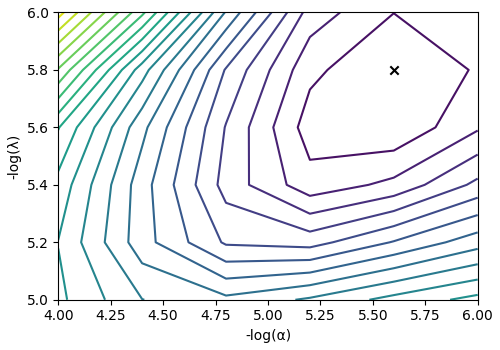### The optimum solution¶

The f attribute of the instance holds the solution.

f_sol = s_lasso_cv.f


The corresponding plot of the solution, along with the true tensor distribution, is shown below.

_, ax = plt.subplots(1, 2, figsize=(9, 3.5), subplot_kw={"projection": "csdm"})

# the plot of the tensor distribution solution.
plot2D(ax, f_sol / f_sol.max(), title="Optimum distribution")

# the plot of the true tensor distribution.
plot2D(ax, true_data_object, title="True distribution")
plt.tight_layout()
plt.show()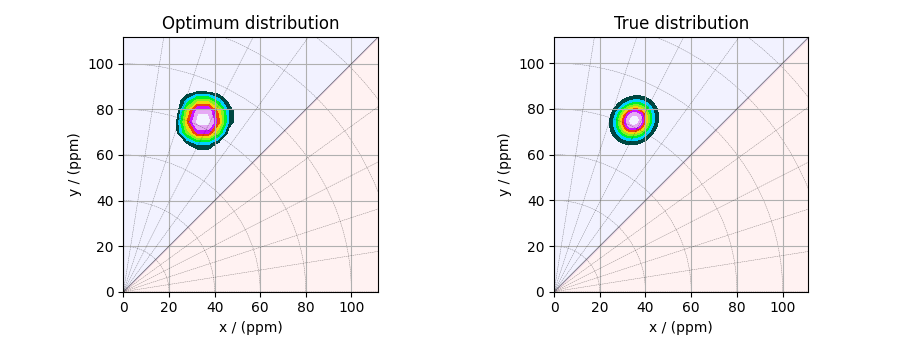Total running time of the script: ( 0 minutes 23.680 seconds)

Gallery generated by Sphinx-Gallery